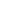# Free CBSE Class 8 Maths Algebraic Expressions And Identities Worksheets

Download free printable Algebraic Expressions And Identities Worksheets to practice. With thousands of questions available, you can generate as many Algebraic Expressions And Identities Worksheets as you want.

## Sample CBSE Class 8 Maths Algebraic Expressions And Identities Worksheet Questions

1.

Using (h + a) (h + b) = h2 + (a + b) h + ab, find 9.8× 9.7

2.

Using (h + a) (h + b) = h2 + (a + b) h + ab, find 98 × 103

3.

Using (h + a) (h + b) = h2 + (a + b) h + ab, find 104 × 103

4.

Using (h + a) (h + b) = h2 + (a + b) h + ab, find 5.2 × 5.1

5.

Using a2 – b2 = (a + b) (a – b), find 153Sq – 147Sq

6.

Using a2 – b2 = (a + b) (a – b), find 12.1Sq – 7.9Sq

7.

Using a2 – b2 = (a + b) (a – b), find 51Sq – 49sq

8.

Using a2 – b2 = (a + b) (a – b), find (1.02)2 – (0.98)2

9.

Show that: (4pq + 3q)2 – (4pq – 3q)2 = 48pq2

10.

Show that: (a – b) (a + b) + (b – c) (b + c) + (c – a) (c + a) = 0

## Find more Algebraic Expressions And Identities Worksheets

Worksheets by UrbanPro

Our worksheets are designed to help students explore various topics, practice skills and enrich their subject knowledge, to improve their academic performance. Designed by Experts who have extensive experience and expertise in teaching a subject, these worksheets will improve your child's problem-solving skills and subject knowledge in a fun and interactive manner.
Check out our free customized worksheets across school boards, grades, subjects and levels of subject knowledge. You can download, print and share these worksheets with anyone, anywhere, anytime!

Get a custom worksheet to practice!

Select your topic & see the magic.

subjectSelect Chapter(s)

Chapters & Subtopics